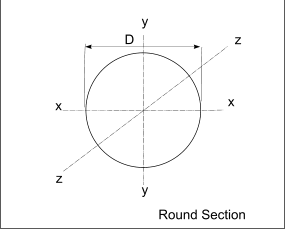Steel Section Index

Simple round Bar SectionsTable of Dimensions + Properties

The table includes various useful properties of steel round bar.    The properties are based on the following

Density of mild steel = 7830 kg/m3
2nd Moment of Area ( Ixx = Iyy ) for a round section = πD4 /64 (Used for bending equations )
Polar moment of Area ( Izz ) for a round section = πD4 /32 (used for torsion equations )
Radius of Gyration ( k ) = R /(2) = (D/2) / (2)
Mass Polar Moment of inertia ( Jm ) = m.k2 (used for shaft/ flywheel dynamic inertia calcs

Note: The mass properties provided can easily be converted to another material by mulipling the mass/mm by the ratio of the density of the chosen material to that of steel.

m copper = msteel . [ Densitycopper / Density steel ]

Jm_copper = Jm_steel . [ Densitycopper / Density steel ]

Example 50mm diameter
CSA = 1963mm2
Weight/mm = 0,01571 kg
2nd moment of Area (Ixx = Iyy )= 0,3068 x 106 mm4
Polar M of Area(Izz ) = 0,6136 x 106 mm4